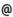## Babes-Bolyai University of Cluj-Napoca Faculty of Mathematics and Computer Science Study Cycle: Graduate SUBJECT

 Code Subject MMA0003 Mathematical Analysis 2 (Calculus in R^n)
 Section Semester Hours: C+S+L Category Type Mathematics 2 3+3+0 fundamental compulsory Mathematics and Computer Science 2 3+3+0 fundamental compulsory Applied Mathematics 2 3+3+0 fundamental compulsory
 Teaching Staff in Charge
 Lect. TRIF Tiberiu Vasile, Ph.D.,  ttrifmath.ubbcluj.roLect. FINTA Zoltan, Ph.D.,  fzoltanmath.ubbcluj.roProf. BRECKNER Wolfgang, Ph.D.,  brecknermath.ubbcluj.ro
 Aims Getting to know the Riemann-Stieltjes integral, the improper integrals, the topology of the Euclidean space R^n, and the differential calculus of functions of several real variables.
 Content 1. The Riemann-Stieltjes integral. Definition of the Riemann-Stieltjes integral. Computation of Riemann-Stieltjes integrals: integration by parts, linearity with respect to the first function and with respect to the second function, change of variable in the Riemann-Stieltjes integral. Criteria of Riemann-Stieltjes integrability with respect to an arbitrary function: Heine's criterion and Cauchy's criterion. Aditivity of the Riemann-Stieltjes integral with respect to the interval. Darboux' criterion of Riemann-Stieltjes integrability of a bounded function with respect to a nondecreasing one. Computation of Riemann-Stieltjes integrals by reduction to Riemann integrals. 2. Real-valued functions of bounded variation. The notion of a function of bounded variation. Classes of functions of bounded variation. Jordan's theorem. Riemann-Stieltjes integrability of a continuous function with respect to a function of bounded variation. 3. Improper integrals. Definition of improper integrals over different types of noncompact intervals. Improper integrals vs Riemann integrals. Computation of improper integrals: linearity, Leibniz-Newton formula, integration by parts, change of variable in improper integrals. Convergence tests for improper integrals. 4. Topology of the Euclidean space R^n. The Euclidian space R^n. Sequences in R^n. Compact sets in R^n. Limits of vector functions of vector variable. Continuity of vector functions of vector variable. 5. Differential calculus in R^n. Linear mappings, the norm of a linear mapping, bijective linear mappings. Derivative of a vector function of a real variable. Differentiability of vector functions of vector variable, directional derivatives, partial derivatives and their connection with differentiability. Operations with differentiable functions, differentiability of the inverse of a bijective function. Mean-value theorems for differentiable functions of vector variable. Functions of the class C^1. The inverse function theorem. Differentiable implicit functions. Optimization problems having equations as constraints. Second order partial derivatives, theorems of Schwarz and Young. The second order differential. Necessary and sufficient optimality conditions. Higher order partial derivatives, Taylor formula.
 References l. BALÁZS M.: Matematikai analizis, Erdélyi Tankönyvtanács, Kolozsvár, 2000 2. BALÁZS M., KOLUMBÁN I.: Matematikai analizis, Dacia Könyvkiado, Kolozsvár-Napoca, 1978 3. BRECKNER W. W.: Analiza matematica. Topologia spatiului R^n. Universitatea din Cluj-Napoca, 1985 4. BROWDER A.: Mathematical Analysis. An Introduction, Springer-Verlag, New York, 1996 5. BUCUR G., CÂMPU E., GÃINÃ S.: Culegere de probleme de calcul diferential si integral, Vol. II, Editura Tehnica Bucuresti 1966. Vol. III, Editura Tehnicã, Bucuresti, 1967 6. COBZAS ST.: Analizã matematicã (Calcul diferential), Presa Universitarã Clujeanã, Cluj-Napoca, 1997 7. DEMIDOVICI B.P.: Culegere de probleme si exercitii de analizã matematicã, Editura Tehnicã, Bucuresti, 1956 8. HEUSER H.: Lehrbuch der Analysis, Teil 1, 11. Auflage, B. G. Teubner, Stuttgart, 1994; Teil 2, 9. Auflage, B. G. Teubner, Stuttgart, 1995 9. MEGAN M.: Bazele analizei matematice, Vol. I + Vol. II, Editura EUROBIT, Timisoara, 1997. Vol. III, Editura EUROBIT, Timisoara, 1998 10. RUDIN W.: Principles of Mathematical Analysis, 2nd Edition, McGraw-Hill, New York, 1964 11. WALTER W.: Analysis, I, II, Springer-Verlag, Berlin, 1990 12. TRIF T.: Probleme de calcul diferential si integral în R^n, Casa Cartii de Stiinta, Cluj-Napoca, 2003
 Assessment A test paper during the semester (20%) + Exam (80%).
 Links: Syllabus for all subjects Romanian version for this subject Rtf format for this subject